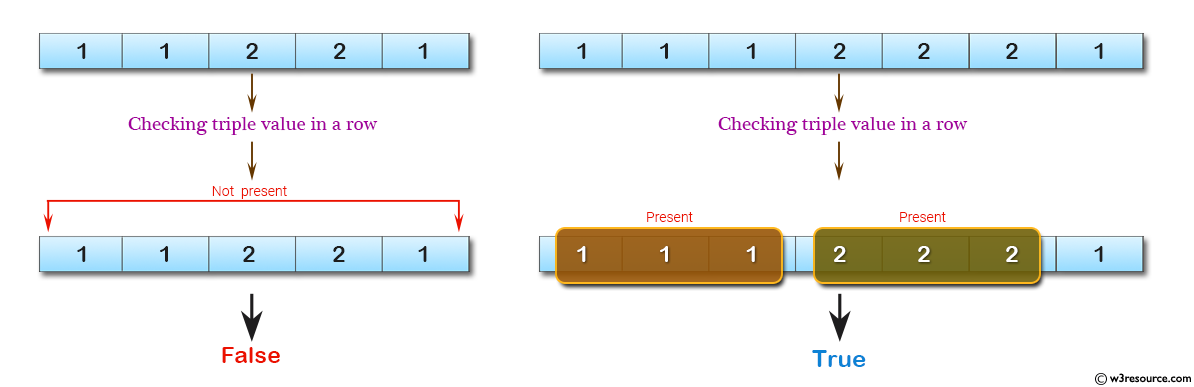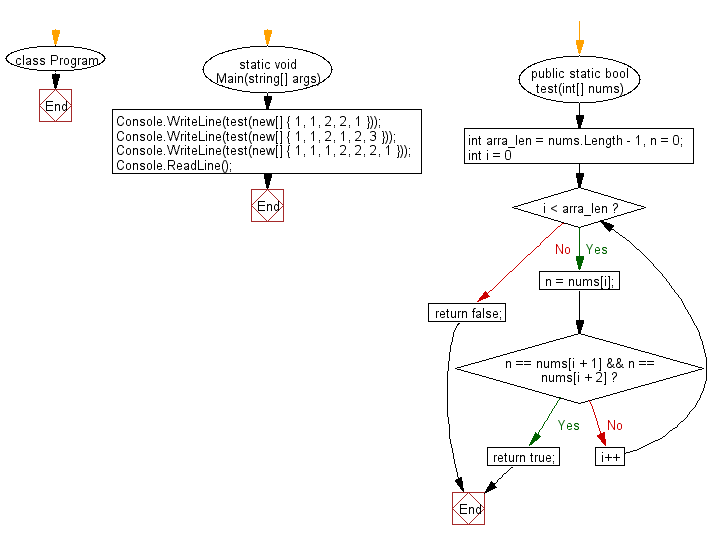﻿ C# - Check if a triple is in an array of integers# C# Sharp Basic Algorithm Exercises: Check whether a triple is presents in an array of integers or not

## C# Sharp Basic Algorithm: Exercise-39 with Solution

Write a C# Sharp program to check whether a triple is presents in an array of integers or not. If a value appears three times in a row in an array it is called a triple.

Pictorial Presentation:Sample Solution:-

C# Sharp Code:

``````using System;
using System.Linq;
namespace exercises
{
class Program
{
static void Main(string[] args)
{
Console.WriteLine(test(new[] { 1, 1, 2, 2, 1 }));
Console.WriteLine(test(new[] { 1, 1, 2, 1, 2, 3 }));
Console.WriteLine(test(new[] { 1, 1, 1, 2, 2, 2, 1 }));
}
public static bool test(int[] nums)
{
int arra_len = nums.Length - 1, n = 0;
for (int i = 0; i < arra_len; i++)
{
n = nums[i];
if (n == nums[i + 1] && n == nums[i + 2]) return true;
}
return false;
}
}
}
```
```

Sample Output:

```False
False
True
```

Flowchart:C# Sharp Code Editor:

Improve this sample solution and post your code through Disqus

What is the difficulty level of this exercise?

Test your Programming skills with w3resource's quiz.

﻿